## Introduction

Dynamic regression models are extension to basic linear regression models where instead of constant but unknown regression coefficients, the underlying coefficients are assumed to vary over “time” according to random walk. In their simplest form these models can be used to model regression models with additional time series component, and they also allow robust modelling of phenomenas where the effect size of the predictor variables can vary during the period of the study. The R (R Core Team 2017) package walker provides an efficient method for fully Bayesian inference of such models, where the main computations are performed using the Markov chain Monte Carlo (MCMC) algorithms provided by Stan (Stan Development Team 2016b, @rstan). This also allows the use of many general diagnostic and graphical tools provided by several Stan related R packages such as ShinyStan (Stan Development Team 2017).

More specifically, the dynamic linear regression model is defined as \begin{aligned} y_t &= x'_t \beta_t + \epsilon_t, \quad t = 1,\ldots, n\\ \beta_{t+1} &= \beta_t + \eta_t, \end{aligned} where $$y_t$$ is the observation at time $$t$$, $$x_t$$ contains the corresponding predictor variables, $$\beta_t$$ is a $$k$$ dimensional vector of regression coefficients at time $$t$$, $$\epsilon_t \sim N(0, \sigma^2_{\epsilon})$$, and $$\eta_t \sim N(0, D)$$, with $$D$$ being $$k \times k$$ diagonal matrix with diagonal elements $$\sigma^2_{i,\eta}$$, $$i=1,\ldots,k$$. Denote the unknown parameters of the model by $$\beta = (\beta'_1, \ldots, \beta'_n)'$$ and $$\sigma = (\sigma_{\epsilon}, \sigma_{1, \eta}, \ldots, \sigma_{k, \eta})$$. We define priors for first $$\beta_1$$ as $$N(\mu_{\beta_1}, \sigma_{\beta_1})$$, and for $$\sigma_i \sim N(\mu_{\sigma_i}, \sigma_{\sigma_i})$$, $$i=1,\ldots,k+1$$, truncated to positive values, with slighly awful notation.

Although in principle writing dynamic linear regression model as above in Stan or BUGS (Lunn et al. 2000) language is straighforward, these standard implementations are computationally inefficient and prone to severe problems related to convergence of the underlying MCMC algorithm. For example in (block) Gibbs sampling approach we target the joint posterior $$p(\beta, \sigma | y)$$ by sampling from $$p(\beta | \sigma, y)$$ and $$p(\sigma | \beta, y)$$. But because of strong autocorrelations between the coeffients $$\beta$$ at different time points, as well as with the associated standard error parameters, this MCMC scheme can be very inefficient. Also, the total number of parameters to sample increase with number of data points $$n$$. Although the Hamiltonian Monte Carlo algoritms offered by Stan are typically more efficient exploring the posterior than Gibbs-type algorithms, we still encounter similar problems (see the illustration in next Section).

An alternative solution used by walker is based on the marginalization of the regression coefficients $$\beta$$ during the MCMC sampling by using the Kalman filter. This provides fast and accurate inference of marginal posterior $$p(\sigma | y)$$. Then, the corresponding joint posterior $$p(\sigma, \beta | y) = p(\beta | \sigma, y)p(\sigma | y)$$ can be obtained by simulating the regression coefficients given marginal posterior of standard deviations. This sampling can be performed for example by simulation smoothing algorithm by Durbin and Koopman (2002). Note that we have opted to sample the $$\beta$$ parameters given $$\sigma$$’s, but it is also possible to obtain somewhat more accurate summary statistics such as mean and variance of these parameters by using the standard Kalman smoother for compution of $$\textrm{E}(\beta| \sigma, y)$$ and $$\textrm{Var}(\beta| \sigma, y)$$, and using the law of total expectation.

In this vignette we first introduce the basic use using simple linear regression model with first order random walk, and then discuss extensions to second order random walk, as well as how to deal with non-Gaussian data.

## Illustration

Let us consider a observations $$y$$ of length $$n=250$$, generated by random walk (i.e. time varying intercept) and two predictors. This is rather small problem, but it was chosen in order to make possible comparisons with the “naive” Stan implementation. For larger problems (in terms of number of observations and especially number of predictors) it is very difficult to get naive implementation to work properly, as even after tweaking several parameters of the underlying MCMC sampler, one typically ends up with divergent transitions or low BMFI index, meaning that the results are not to be trusted.

First we simulate the coefficients and the predictors:

set.seed(1)
n <- 100
beta1 <- cumsum(c(0.5, rnorm(n - 1, 0, sd = 0.05)))
beta2 <- cumsum(c(-1, rnorm(n - 1, 0, sd = 0.15)))
x1 <- rnorm(n, mean = 2)
x2 <- cos(1:n)
rw <- cumsum(rnorm(n, 0, 0.5))
ts.plot(cbind(rw, beta1 * x1, beta2 * x2), col = 1:3)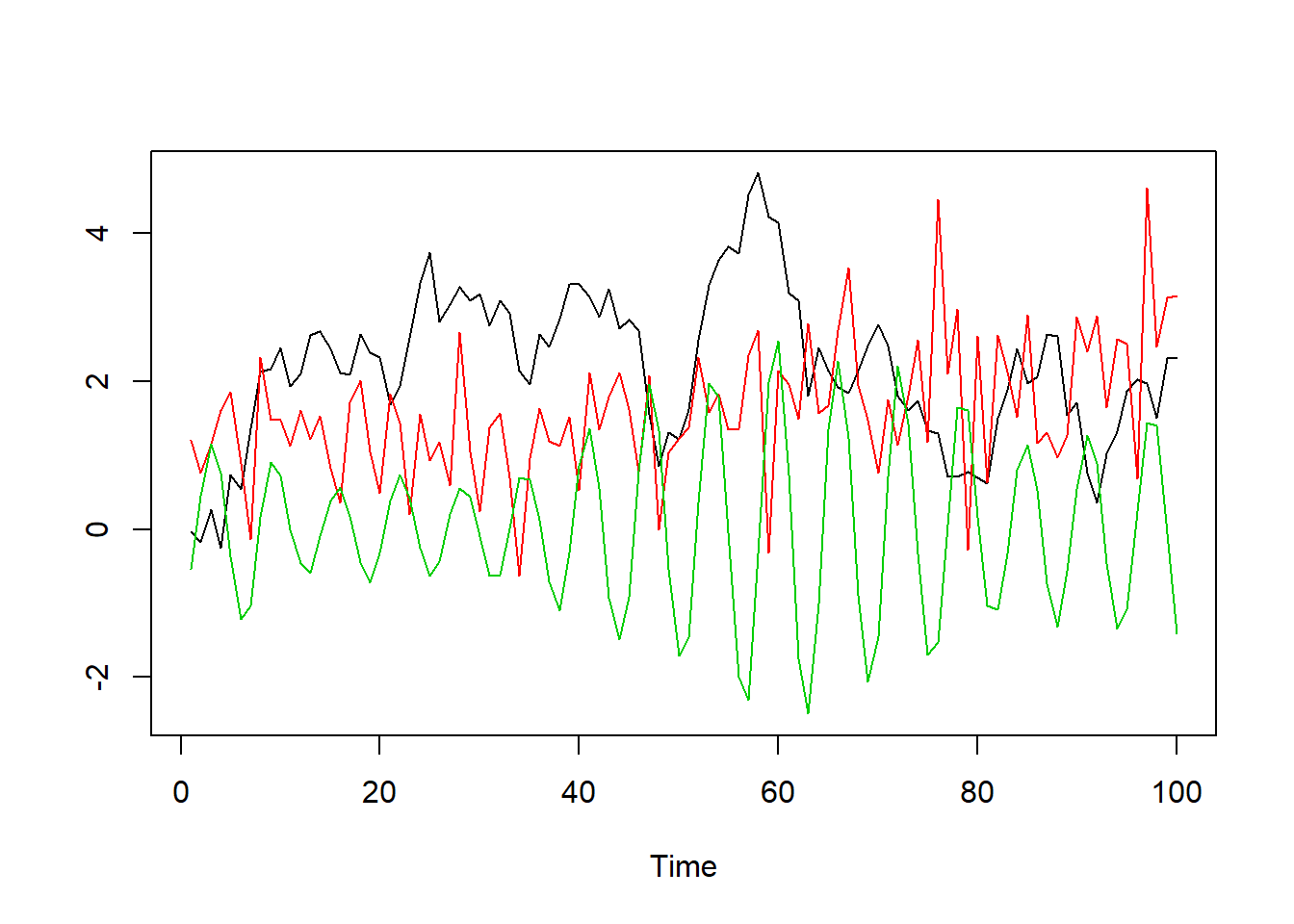signal <- rw + beta1 * x1 + beta2 * x2
y <- rnorm(n, signal, 0.5)
ts.plot(cbind(signal, y), col = 1:2)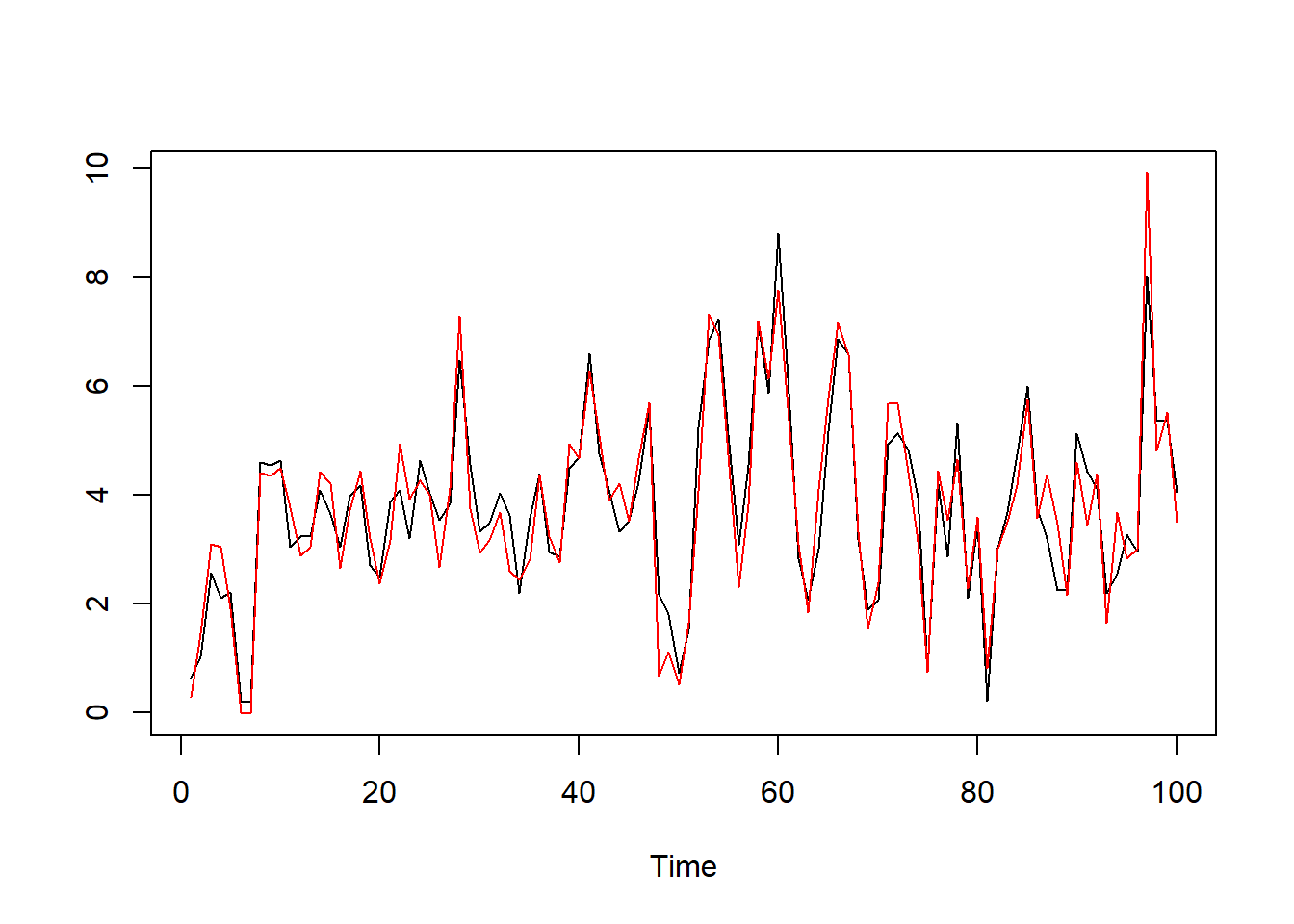Then we can call function walker. The model is defined as a formula like in lm, and we can give several arguments which are passed to sampling method of rstan, such as number of iteration iter and number of chains chains (default values for these are 2000 and 4). In addition to these, we use arguments beta_prior and sigma_prior, which define the prior distributions for $$\beta_1$$ and $$\sigma$$ respectively. These arguments should length two vectors, where the first value defines the prior mean, and the second value defines the prior standard deviation.

fit <- walker(y ~ -1 + rw1(~ x1 + x2, beta_prior = c(0, 10), sigma_prior = c(0, 10)),
refresh = 0, chains = 2, sigma_y_prior = c(0, 10))

We sometimes get a few (typically one) warning message about numerical problems, as the sampling algorithm warms up, but this is nothing to be concerned with (if more errors occur, then a Github issue for walker package is more than welcome).

The output of walker is walker_fit object, which is essentially a list with stanfit from Stan’s sampling function, and the original observations y and the covariate matrix xreg. Thus we can use all the ready made tools for postprocessing stanfit objects:

print(fit$stanfit, pars = c("sigma_y", "sigma_rw1")) ## Inference for Stan model: walker_lm. ## 2 chains, each with iter=2000; warmup=1000; thin=1; ## post-warmup draws per chain=1000, total post-warmup draws=2000. ## ## mean se_mean sd 2.5% 25% 50% 75% 97.5% n_eff Rhat ## sigma_y 0.49 0.01 0.14 0.15 0.41 0.51 0.59 0.74 609 1 ## sigma_rw1 0.60 0.00 0.13 0.37 0.50 0.59 0.68 0.90 741 1 ## sigma_rw1 0.08 0.00 0.04 0.01 0.05 0.08 0.10 0.17 1173 1 ## sigma_rw1 0.31 0.00 0.10 0.15 0.24 0.30 0.37 0.56 1142 1 ## ## Samples were drawn using NUTS(diag_e) at Thu Jan 23 13:19:58 2020. ## For each parameter, n_eff is a crude measure of effective sample size, ## and Rhat is the potential scale reduction factor on split chains (at ## convergence, Rhat=1). mcmc_areas(as.matrix(fit$stanfit), regex_pars = c("sigma_y", "sigma_rw1"))
## Warning: expand_scale() is deprecated; use expansion() instead.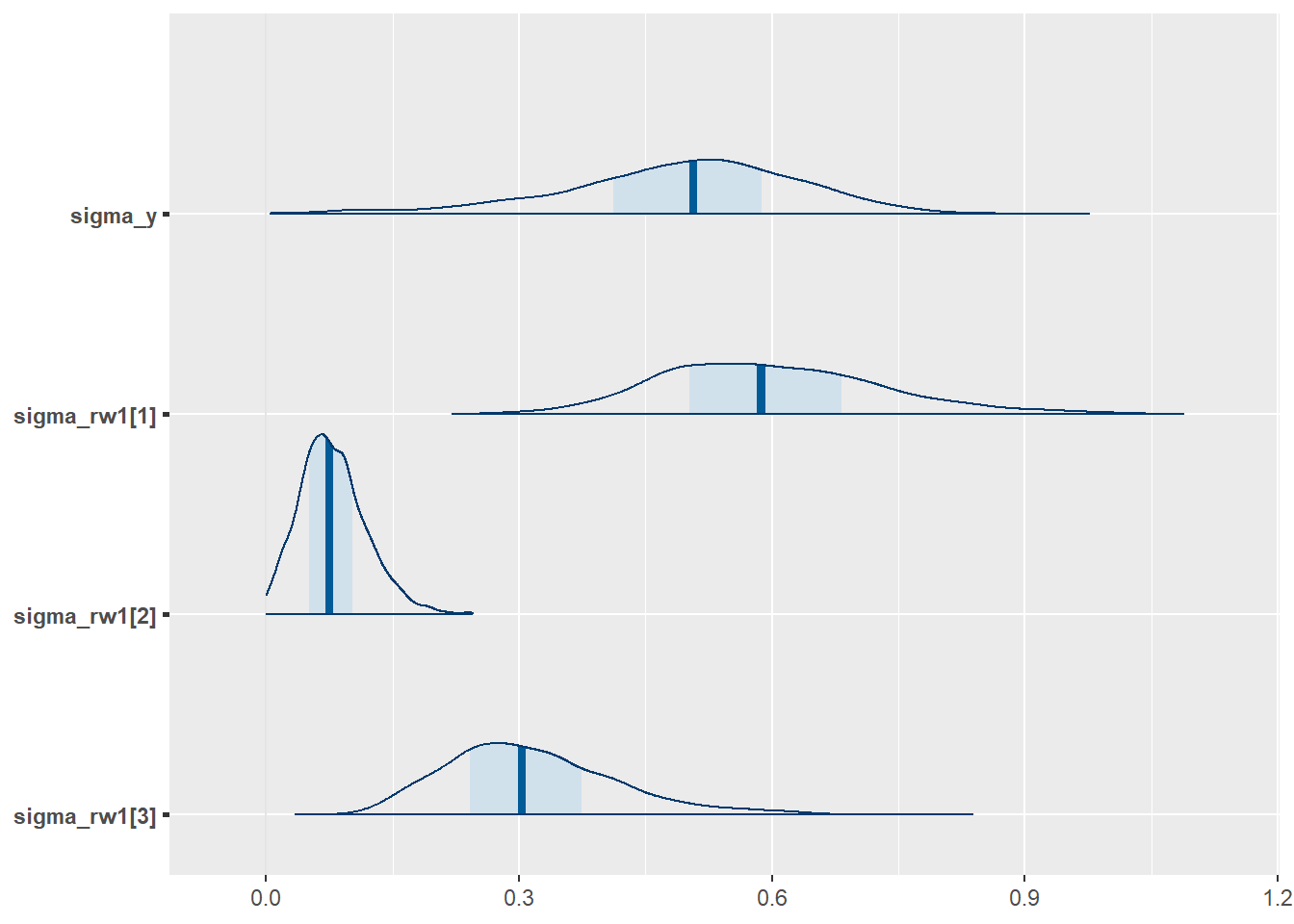Let’s check how well our estimates of $$\beta$$ coincide with the true values (the solid lines correspond to true values):

betas <- summary(fit$stanfit, "beta_rw")$summary[, "mean"]

ts.plot(cbind(rw, beta1, beta2, matrix(betas, ncol = 3)),
col = rep(1:3, 2), lty = rep(1:2, each = 3))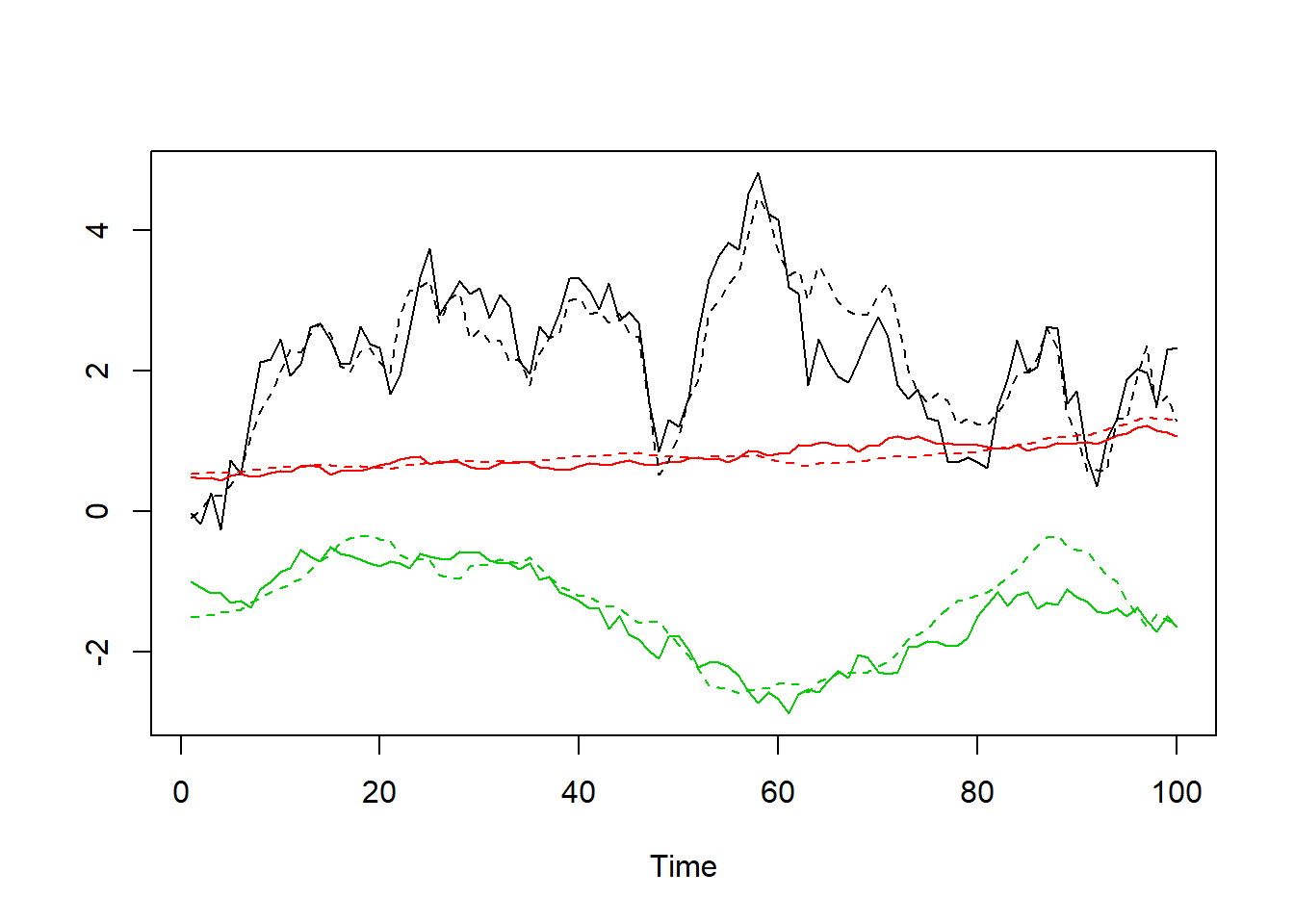There is also simpler (and prettier) ggplot based plotting function for coefficients:

plot_coefs(fit)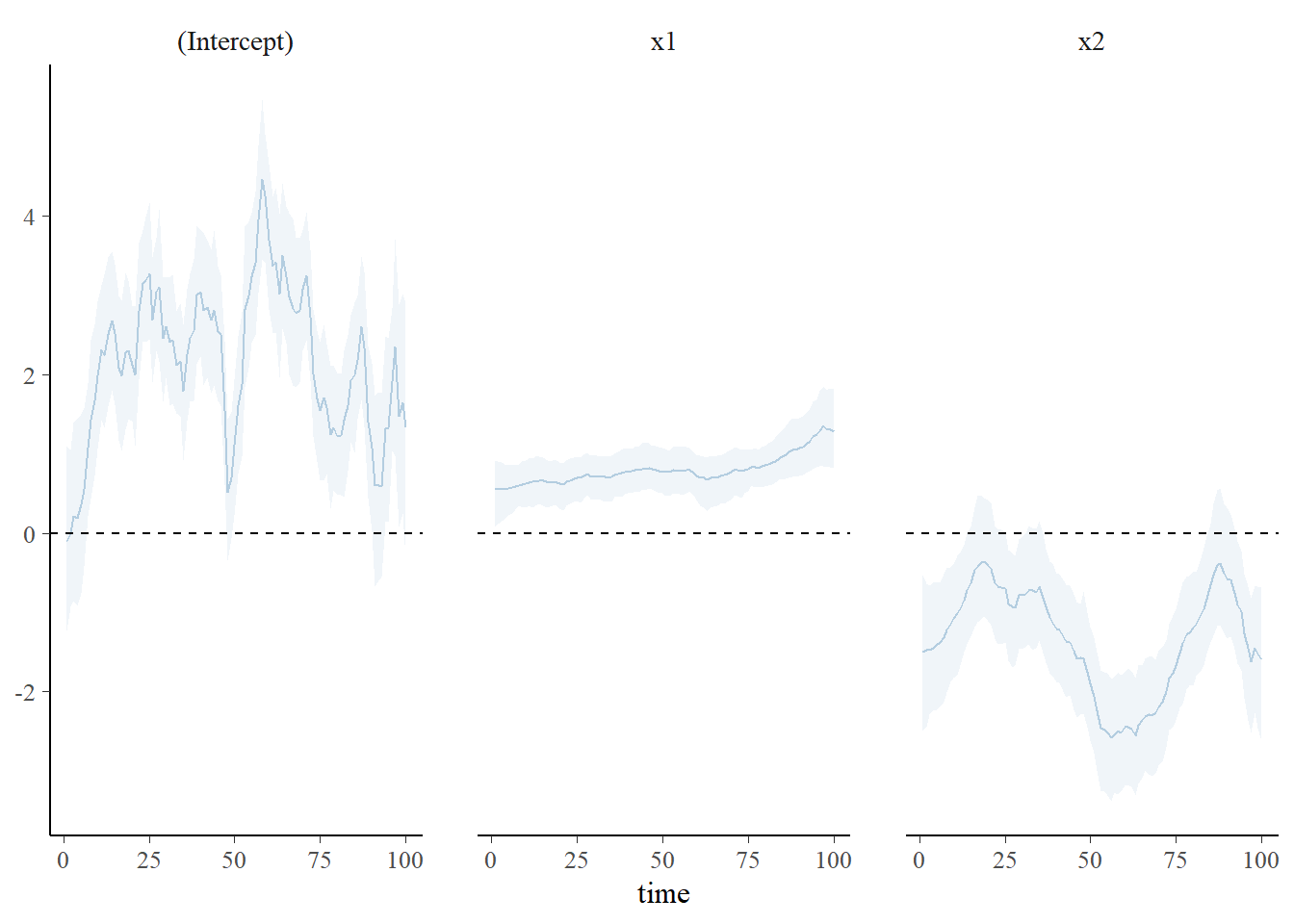### Posterior predictive checks

The walker function also returns samples from the posterior predictive distribution $$p(y^{\textrm{rep}} | y) = \int p(y^{\textrm{rep}} | \beta, \sigma, y) p(\beta, \sigma | y) \textrm{d}\beta\textrm{d}\sigma$$. This can be used to used for example in assessment of model fit to the data. By comparing the replication series (mean and 95% quantiles in black) and the original observations (in red) we see that very good overlap, which is not that suprising given that we know the correct model:

pp_check(fit)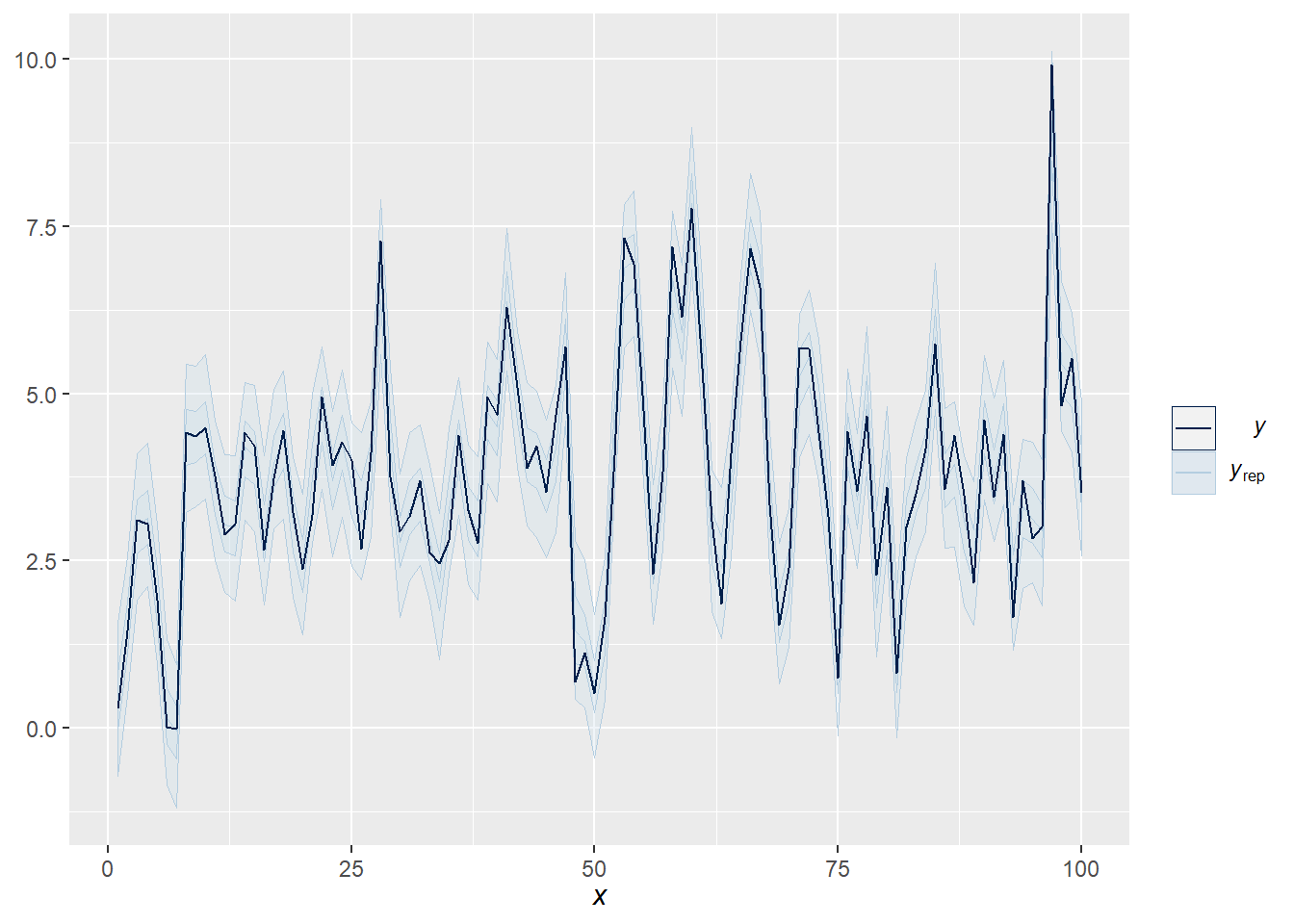### Out-of-sample prediction

It is also possible to obtain actual forecasts given new covariates $$x^{new}$$:

new_data <- data.frame(x1 = rnorm(10, mean = 2), x2 = cos((n + 1):(n + 10)))
pred <- predict(fit, new_data)
plot_predict(pred)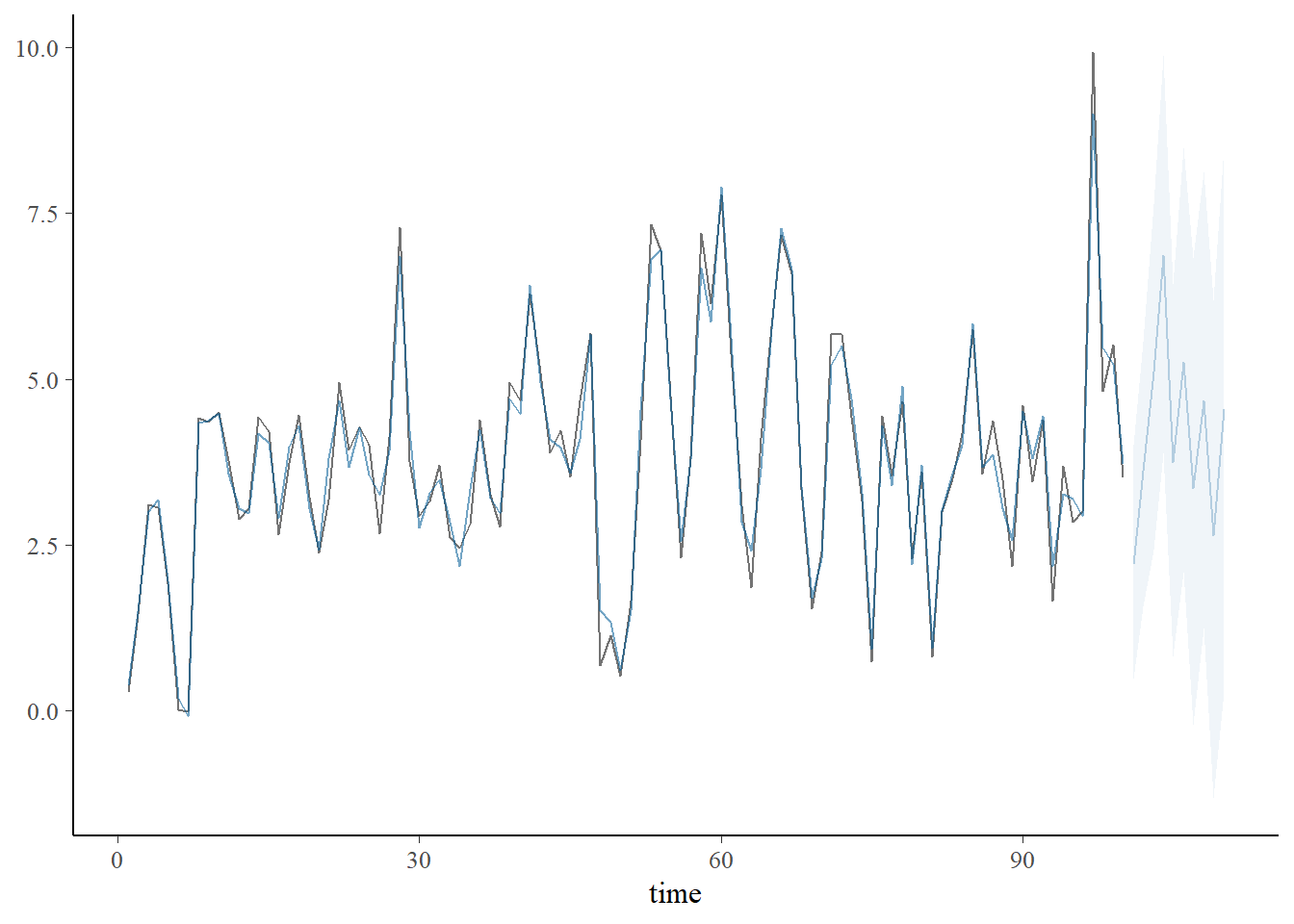## Extensions: Smoother effects and non-Gaussian models

When modelling regression coefficients as a simple random walk, the posterior estimates of these coefficients can have large short-term variation which might not be realistic in practice. One way of imposing more smoothness for the estimates is to switch from random walk coefficients to integrated second order random walk coefficients: $\beta_{t+1} = \beta_t + \nu_t,\\ \nu_{t+1} = \nu_t + \eta_t.$ This is essentially local linear trend model (Harvey 1989) with restriction that there is no noise on the $$\beta$$ level. This model can be estimated by switching rw1 function inside of the walker formula to rw1, with almost identical interface, but now $$\sigma$$ correspond to the standard deviations of the slope terms $$\nu$$. The prior for $$\nu$$ most also be defined. Using RW2 model, the coefficient estimates of our example model are clearly smoother:

fit_rw2 <-walker(y ~ -1 +
rw2(~ x1 + x2, beta_prior = c(0, 10), sigma_prior = c(0, 10), slope_prior = c(0, 10)),
refresh = 0, chains = 2, sigma_y_prior = c(0, 10))
plot_coefs(fit_rw2)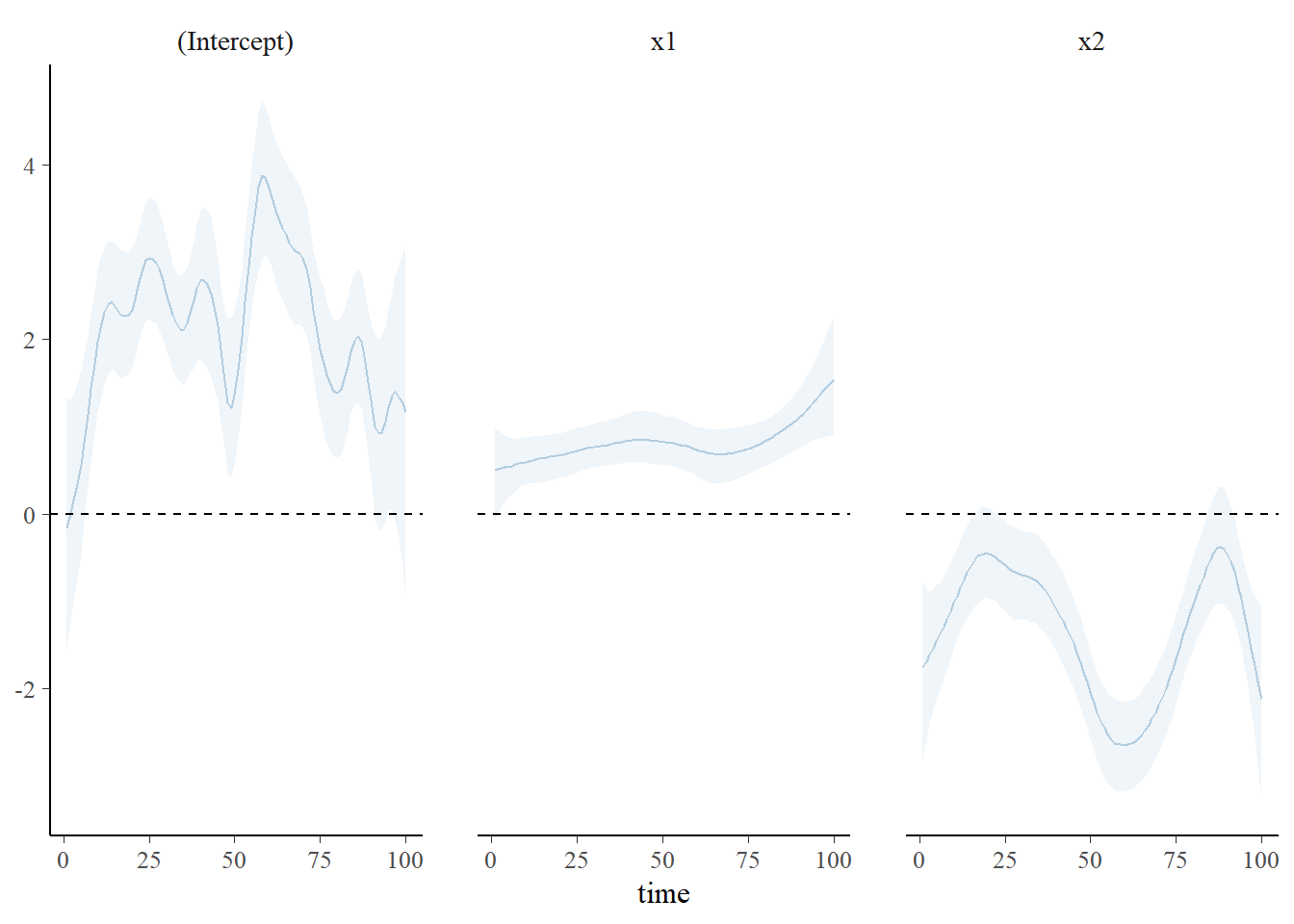So far we have focused on simple Gaussian linear regression. The above treatment cannot be directly extended to non-Gaussian models such as Poisson regression, as the marginal log-likelihood is intractable. However, it is possible to use relatively accurate Gaussian approximations, and the resulting approximate posterior can then be weighted using importance sampling type correction (Vihola, Helske, and Franks 2016), leading to exact inference. Function walker_glm extends the the package to handle Poisson and binomial observations using the aforementioned methodology.

## Comparison with naive approach

We now compare the efficiency of the “naive” implementation and the state space approach. For this, we use function walker_rw1, which supports only basic random walk models. We can perform the same analysis with naive implementation by setting the argument naive to TRUE:

naive_walker <- walker_rw1(y ~ x1 + x2, seed = 1, refresh = 0, chains = 2,
beta_prior = cbind(0, rep(5, 3)), sigma_prior = cbind(0, rep(2, 4)),
naive = TRUE, control = list(adapt_delta = 0.95, max_treedepth = 15))
## Warning: There were 22 divergent transitions after warmup. Increasing adapt_delta above 0.95 may help. See
## http://mc-stan.org/misc/warnings.html#divergent-transitions-after-warmup
## Warning: Examine the pairs() plot to diagnose sampling problems
## Warning: Bulk Effective Samples Size (ESS) is too low, indicating posterior means and medians may be unreliable.
## Running the chains for more iterations may help. See
## http://mc-stan.org/misc/warnings.html#bulk-ess
## Warning: Tail Effective Samples Size (ESS) is too low, indicating posterior variances and tail quantiles may be unreliable.
## Running the chains for more iterations may help. See
## http://mc-stan.org/misc/warnings.html#tail-ess
print(naive_walker$stanfit, pars = c("sigma_y", "sigma_b")) ## Inference for Stan model: rw1_model_naive. ## 2 chains, each with iter=2000; warmup=1000; thin=1; ## post-warmup draws per chain=1000, total post-warmup draws=2000. ## ## mean se_mean sd 2.5% 25% 50% 75% 97.5% n_eff Rhat ## sigma_y 0.51 0.01 0.12 0.23 0.44 0.51 0.59 0.74 71 1.03 ## sigma_b 0.58 0.01 0.11 0.36 0.50 0.58 0.66 0.81 145 1.01 ## sigma_b 0.08 0.00 0.04 0.01 0.05 0.08 0.10 0.17 436 1.01 ## sigma_b 0.32 0.01 0.11 0.15 0.25 0.31 0.38 0.56 250 1.01 ## ## Samples were drawn using NUTS(diag_e) at Thu Jan 23 13:22:52 2020. ## For each parameter, n_eff is a crude measure of effective sample size, ## and Rhat is the potential scale reduction factor on split chains (at ## convergence, Rhat=1). Let’s run the state space version again: kalman_walker <- walker_rw1(y ~ x1 + x2, seed = 1, refresh = 0, chains = 2, beta_prior = cbind(0, rep(5, 3)), sigma_prior = cbind(0, rep(2, 4)), naive = FALSE) print(kalman_walker$stanfit, pars = c("sigma_y", "sigma_b"))
## Inference for Stan model: rw1_model.
## 2 chains, each with iter=2000; warmup=1000; thin=1;
## post-warmup draws per chain=1000, total post-warmup draws=2000.
##
##            mean se_mean   sd 2.5%  25%  50%  75% 97.5% n_eff Rhat
## sigma_y    0.49    0.01 0.14 0.16 0.41 0.50 0.58  0.72   577    1
## sigma_b 0.60    0.00 0.13 0.38 0.51 0.59 0.69  0.89   748    1
## sigma_b 0.08    0.00 0.04 0.01 0.05 0.07 0.10  0.16   976    1
## sigma_b 0.31    0.00 0.10 0.15 0.25 0.30 0.37  0.53  1481    1
##
## Samples were drawn using NUTS(diag_e) at Thu Jan 23 13:23:09 2020.
## For each parameter, n_eff is a crude measure of effective sample size,
## and Rhat is the potential scale reduction factor on split chains (at
## convergence, Rhat=1).
sum(get_elapsed_time(kalman_walker$stanfit)) ##  15.468 sum(get_elapsed_time(naive_walker$stanfit))
##  133.982

With naive implementation we get smaller effective sample sizes and much higher computation time, as well as some indications of divergence problems, even with adjusted step size (argument adapt_delta).

## Discussion

In this vignette we illustrated the benefits of marginalisation in the context of dynamic regression models. The underlying idea is not new; this approach is typical especially in classic Metropolis-type algorithms for linear-Gaussian state space models where the marginal likelihood $$p(y | \theta)$$ (where $$\theta$$ denotes the hyperparameters i.e. not the latents states such as $$\beta$$’s in current context) is used in the computation of the acceptance probability. Here instead of building specific MCMC machinery, we rely on readily available Hamiltonian Monte Carlo based Stan software, thus allowing us to enjoy the benefits of diverse tools of the Stan community.

The motivation behind the walker was to test the efficiency of importance sampling type weighting method of Vihola, Helske, and Franks (2016) in a dynamic generalized linear model setting within the HMC context. Although some of our other preliminary results have suggested that naive combination of the HMC with IS weighting can provide rather limited computational gains, in case of dynamic GLMs so called global approximation technique (Vihola, Helske, and Franks 2016) provides fast and robust alternative to standard HMC approach of Stan.

In the future, the prior definitions and some of the post-processing options will extended to allow more flexible modelling. Collaboration regarding the development of the package as well as interesting applications are highly welcome.

## Acknowledgements

This work has been supported by the Academy of Finland research grants 284513 and 312605.